Function Repository Resource:

# TableWhile

Create tables of values while given criteria hold

Contributed by: Lukas Lang
 ResourceFunction["TableWhile"][expr,{i,start,condition}] creates a table of expr with i running from start until condition[expr] no longer returns True. ResourceFunction["TableWhile"][expr,{i,condition}] creates a table of expr with i starting at 1. ResourceFunction["TableWhile"][expr,{i,start,condition,step}] increments i in increments of step. ResourceFunction["TableWhile"][expr,condition] creates a list of expr until condition[expr] no longer returns True. ResourceFunction["TableWhile"][expr,{i,…},{j,…},…] uses multiple iterators, each with its own condition. ResourceFunction["TableWhile"][expr,{i,…,{end,condition},…}] stops either when i reaches end or when condition[expr] no longer returns True. ResourceFunction["TableWhile"][expr,{i,…,{condition,listCondition},…}] continues while both condition[expr] and listCondition[{expr1,expr2,…}] return True. ResourceFunction["TableWhile"][expr,{i,…,{end,condition,listCondition},…] continues until i reaches end or either of the conditions fail. ResourceFunction["TableWhile"][expr,{i,{i1,i2,…}},…] uses successive values i1,i2,…. ResourceFunction["TableWhile"][expr,{i,{i1,i2,…},conditions},…] stops iterations when the conditions first fail.

## Details and Options

ResourceFunction["TableWhile"] works like Table, but supports conditions as criteria for termination.
ResourceFunction["TableWhile"] supports an extended version of the standard iterator specification:
 {i,end} run i from 1 to end in increments of 1 {i,start,end} start from start {i,start,end,step} use increments of step {i,{i1,i2,…}} use successive values i1,i2,… {i,{i1,i2,…},conditions} only continue as long as condition is met
In all iterator specifications, end can be one of the following:
 n stop when the iteration variable reaches n condition stop when condition[expr] no longer returns True {condition,listCondition} also stop when listCondition[{expr1,expr2,…}] no longer returns True {None, listCondition} use only listCondition {n, …} also stop when the iteration variable reaches n
ResourceFunction["TableWhile"] uses NumericQ to distinguish between iteration bounds and conditions. This means that unlike for Table, symbolic iteration boundaries are not supported.
In the iterator specification {i,{i1,i2,},conditions}, conditions can be either a single condition or a pair {condition,listCondition}.
In ResourceFunction["TableWhile"][expr,{,condition,}], condition is applied to each expression of the resulting list.
In ResourceFunction["TableWhile"][expr,{,{,listCondition},}], listCondition is applied to the list of expressions generated so far at each step.
ResourceFunction["TableWhile"] terminates when either of condition[] or listCondition[] does not give True.
When condition[expr] in ResourceFunction["TableWhile"][expr,{i,,condition,}] is evaluated, the value of i of the current iteration can also be accessed. The same is true for evaluations of listCondition[{expr1,expr2,}].
ResourceFunction["TableWhile"][expr,spec1,spec2] is effectively equivalent to ResourceFunction["TableWhile"][ResourceFunction["TableWhile"][expr,spec2],spec1].
ResourceFunction["TableWhile"] effectively uses Block to localize iterator variables.
ResourceFunction["TableWhile"] accepts the following options:
 MaxIterations 10000 how many elements to generate at most
As long as only numeric iterators are used, Table[expr,spec1,] is effectively equivalent to ResourceFunction["TableWhile"][expr,spec1,].
As ResourceFunction["TableWhile"] uses Do internally, loop control functions such as Continue, Break, … might affect the result in ways that are not expected.

## Examples

### Basic Examples (3)

Generate all primes less than 100:

 In:=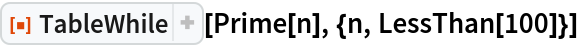Out=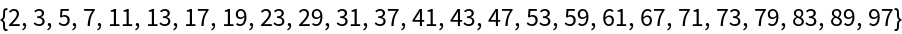Generate all Fibonacci numbers below 1000, where the index has the form 3m+1:

 In:=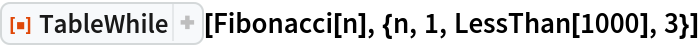Out=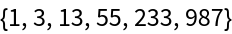Generate numbers of the form nn-2 below a billion:

 In:=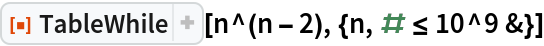Out=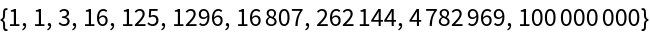Computing an explicit iteration boundary is not possible:

 In:=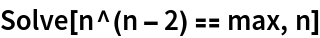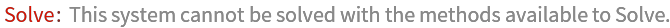Out=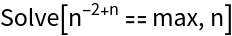### Scope (3)

Generate reciprocals of the counting numbers until their mean falls below 0.1:

 In:=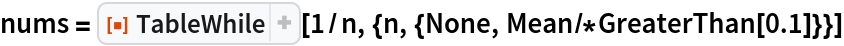Out=Check that the mean is indeed larger than 0.1:

 In:=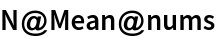Out=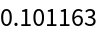Adding the next number would bring the mean below 0.1:

 In:=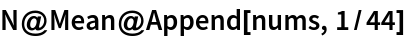Out=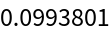Generate random numbers as long as they are below 0.8:

 In:=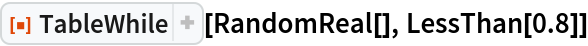Out=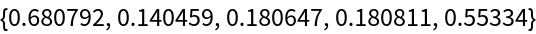Generate several such lists and plot their length distribution:

 In:=Out=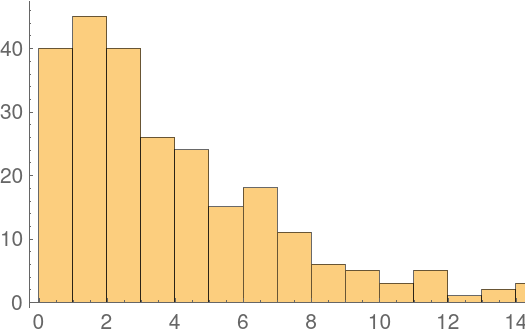Generate polygonal numbers PolygonalNumber[r,n] for each r until their total exceeds 1000, or the individual numbers exceed 200. Stop increasing r when fewer than five numbers are generated:

 In:=Out=### Options (2)

#### MaxIterations (2)

With the default setting MaxIterations10000, only 10,000 elements will be generated:

 In:=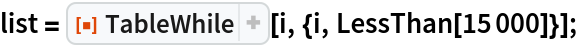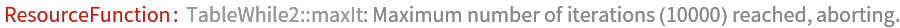In:=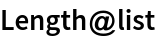Out=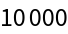Set MaxIterations to a higher value to generate all elements:

 In:=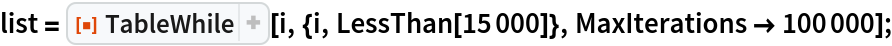In:=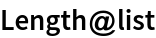Out=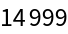### Properties and Relations (1)

The result of TableWhile can often be emulated by generating a sufficiently long list and applying TakeWhile to it. However, this can be inefficient and elements might be missed:

 In:=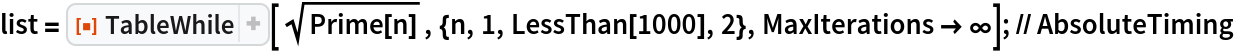Out=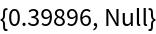In:=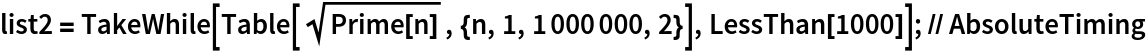Out=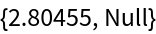### Neat examples (4)

Generate all points in the positive quadrant with integer coordinates that lie in a circle with radius 8:

 In:=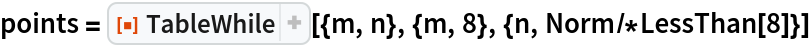Out=Plot the points together with the boundary:

 In:=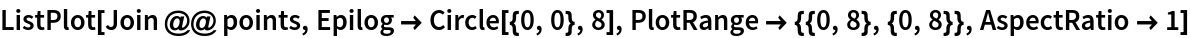Out=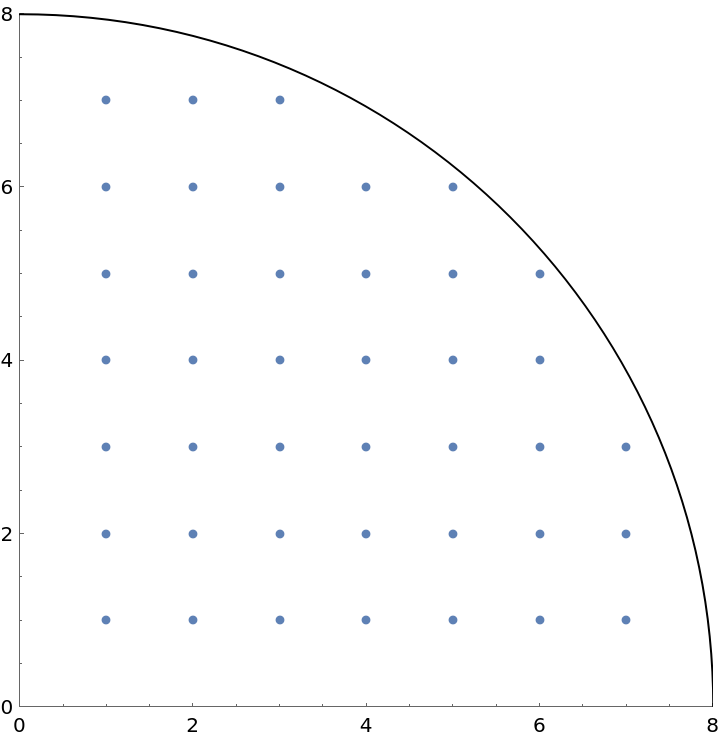Generate the same list of points using a condition involving m and n:

 In:=Out=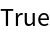Instead of giving an explicit boundary for m, the iteration can also be stopped if there are no longer any valid n:

 In:=Out=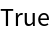## Version History

• 2.0.0 – 06 January 2020
• 1.0.0 – 03 September 2019